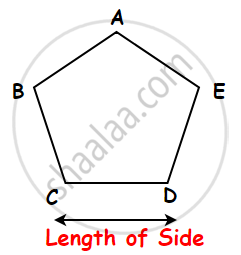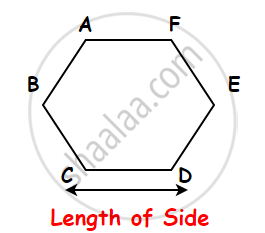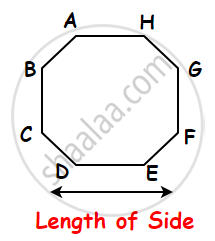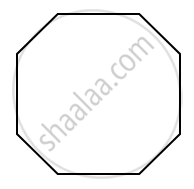# Perimeter of Polygon

#### definition

Polygon: Polygon refers to a closed 2D shape which is made up of a finite number of line segments, but the perimeter is a one-dimensional measurement.

#### formula

The perimeter of a regular polygon = (length of one side) × number of sides.

The perimeter of an Irregular polygon = Sum of all sides of Irregular polygons.

# Perimeter of Polygon:

• Polygon refers to a closed 2D shape which is made up of a finite number of line segments, but the perimeter is a one-dimensional measurement.

• The total distance of the outer sides of a closed figure is known as the perimeter of the polygon. It is the total length of all sides of a polygon.

• The perimeter of Polygon = Sum of all sides

• The unit of the perimeter of any polygon will remain the same as the unit of its respective sides. If the sides are given in different units, then convert them to the same unit and then find the perimeter.

• Perimeter is the distance around a closed figure while area is the part of the plane or region occupied by the closed figure.

## A. The perimeter of a regular polygon:

A regular polygon has all sides equal. Therefore, we can find the perimeter of a regular polygon by repeated addition. So, we add the same length ‘n’ times if there are ‘n’ sides in the polygon.

The perimeter of a regular polygon = (length of one side) × number of sides.

### 1) Perimeter of a Regular pentagon:

Regular pentagons have five congruent sides, five congruent interior angles, and five congruent exterior angles.Perimeter of the Regular Pentagon = Sum of the lengths of its Five sides.

= AB + BC + CD + DE + AE
= AB + AB + AB + AB + AB.....(A regular pentagon has five equal sides.)
= 5 × AB
= 5 × side.

Perimeter of a Regular Pentagon = Side + Side + Side + Side + Side i.e., Perimeter of a Regular Pentagon = 5 × length of a side

### 2) Perimeter of a regular hexagon:

• A hexagon is a six-sided polygon.

• Regular Hexagon has Six congruent sides, six congruent interior angles, and six congruent exterior angles.Perimeter of the Regular hexagon = Sum of the lengths of its Six sides.

= AB + BC + CD + DE + EF + FA
= AB + AB + AB + AB + AB + AB.....(A regular hexagon has six equal sides.)
= 6 × AB
= 6 × side.

Perimeter of a Regular hexagon = Side + Side + Side + Side + Side + Side i.e., Perimeter of a Regular Hexagon = 6 × length of a side.

### 3) Perimeter of a regular octagon:

• A regular octagon is an eight-sided polygon.

• A regular octagon has eight congruent sides, eight congruent interior angles, and eight congruent exterior angles.Perimeter of a regular octagon = Sum of the lengths of its eight sides.

= AB + BC + CD + DE + EF + FG + GH + AH
= AB + AB + AB + AB + AB + AB + AB + AB.....(A regular hexagon has eight equal sides.)
= 8 × AB
= 8 × side.

Perimeter of a regular octagon = Side + Side + Side + Side + Side + Side + Side + Side. i.e., Perimeter of a regular octagon = 8 × length of a side.

### 4) Perimeter of an 'n' sided polygon:

Polygon refers to a closed 2D shape which is made up of a finite number of the line segments.

Perimeter of n sided polygon = n × length of one side.

For Example, Perimeter of Decagon = 10 × length of one side.## B. Perimeter of an irregular polygon:

Irregular polygons are polygons that have unequal angles and unequal sides. It is the total distance around a polygon. It can be found by adding together all the sides of the polygon.The perimeter of an Irregular polygon = Sum of all sides of Irregular polygons.

If you would like to contribute notes or other learning material, please submit them using the button below.

### Shaalaa.com

Perimeter Of A Regular Shape [00:10:29]
S
0%